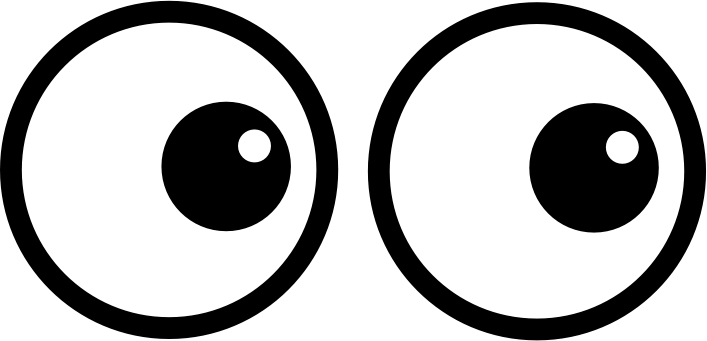# RATL 2 | Lesson 2 | Practice (Adding Fractions)Add the fractions below using two methods:

1. the standard algorithm, and if possible,
2. by factoring the numerator and denominator to find the common denominator:
1. $$\dfrac{3}{8}+\dfrac{1}{6}=$$
2. $$\dfrac{2}{8}+\dfrac{5}{8}=$$
3. $$\dfrac{3}{16}+\dfrac{4}{21}=$$
4. $$\dfrac{1}{3}+\dfrac{1}{5}=$$

Check solutions here.